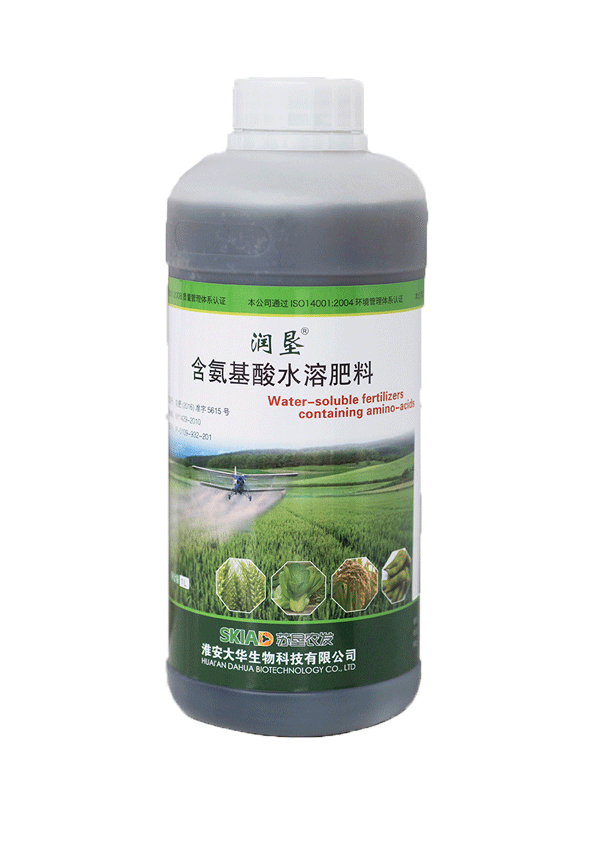• 水产专用
• 大量元素水溶首页
• 含腐殖酸水溶首页
• 含氨基酸水溶首页
• 发酵菌剂
• 沼气发酵菌剂
• 肥水专用
• 净水剂
• 发酵增产剂
• 生物反应堆菌剂
• 秸秆饲料发酵剂
• 酵素菌速腐剂
• 废菌料发酵剂
• 育苗基质
• 水稻育苗基质
• 蓝莓栽培基质
• 蔬菜育苗基质
• 生物有机肥
• 有机首页
• 生物有机肥
• 食用菌专用
• 发酵增产剂
• 菇宝乐
• 联系我们
• 产品分类
• 发酵菌剂
• 生物反应堆菌剂
• 沼气发酵菌剂
• 肥水专用
• 发酵增产剂
• 秸秆饲料发酵剂
• 废菌料发酵剂
• 酵素菌速腐剂
• 净水剂
• 水溶首页
• 大量元素水溶首页
• 含腐殖酸水溶首页
• 含氨基酸水溶首页
• 育苗基质
• 蓝莓栽培基质
• 蔬菜育苗基质
• 水稻育苗基质
• 生物有机肥
• 有机首页
• 生物有机肥
• 水产制剂
• 水产专用
• 食用菌专用
• 菇宝乐
• 发酵增产剂
• 园艺资材
• 有机首页
• 水稻育苗基质
• 花盆
• 含氨基酸水溶首页
• 菇宝乐
• 发酵增产剂
• 土壤修复剂
• 土壤调理剂
• 产品分类
• 发酵菌剂
• 水溶首页
• 育苗基质
• 生物有机肥
• 食用菌专用
• 园艺资材
• 土壤调理剂
• 土壤修复剂
• 水产制剂
• 产品分类
• 土壤调理剂
• 土壤修复剂
• 水产制剂
• 水产专用
• 育苗基质
• 蓝莓栽培基质
• 蔬菜育苗基质
• 水稻育苗基质
• 发酵菌剂
• 发酵增产剂
• 肥水专用
• 沼气发酵菌剂
• 净水剂
• 生物反应堆菌剂
• 秸秆饲料发酵剂
• 废菌料发酵剂
• 酵素菌速腐剂
• 水溶首页
• 大量元素水溶首页
• 含氨基酸水溶首页
• 含腐殖酸水溶首页
• 生物有机肥
• 有机首页
• 生物有机肥
• 食用菌专用
• 菇宝乐
• 发酵增产剂
• 园艺资材
• 菇宝乐
• 发酵增产剂
• 花盆
• 水稻育苗基质
• 含氨基酸水溶首页
• 有机首页
• 产品分类
• 发酵菌剂
• 发酵增产剂
• 沼气发酵菌剂
• 净水剂
• 生物反应堆菌剂
• 秸秆饲料发酵剂
• 废菌料发酵剂
• 酵素菌速腐剂
• 肥水专用
• 水溶首页
• 大量元素水溶首页
• 含腐殖酸水溶首页
• 含氨基酸水溶首页
• 育苗基质
• 蓝莓栽培基质
• 蔬菜育苗基质
• 水稻育苗基质
• 生物有机肥
• 有机首页
• 生物有机肥
• 土壤调理剂
• 食用菌专用
• 菇宝乐
• 发酵增产剂
• 园艺资材
• 有机首页
• 水稻育苗基质
• 花盆
• 含氨基酸水溶首页
• 发酵增产剂
• 菇宝乐
• 土壤修复剂
• 水产制剂
• 水产专用
• 产品分类
• 土壤调理剂
• 土壤修复剂
• 水产制剂
• 水产专用
• 发酵菌剂
• 沼气发酵菌剂
• 肥水专用
• 净水剂
• 发酵增产剂
• 生物反应堆菌剂
• 秸秆饲料发酵剂
• 废菌料发酵剂
• 酵素菌速腐剂
• 水溶首页
• 含氨基酸水溶首页
• 含腐殖酸水溶首页
• 大量元素水溶首页
• 育苗基质
• 蓝莓栽培基质
• 蔬菜育苗基质
• 水稻育苗基质
• 生物有机肥
• 有机首页
• 生物有机肥
• 食用菌专用
• 菇宝乐
• 发酵增产剂
• 园艺资材
• 含氨基酸水溶首页
• 菇宝乐
• 发酵增产剂
• 水稻育苗基质
• 有机首页
• 花盆
• 网站首页
• 关于我们
• 总经理致辞
• 企业文化
• 组织架构
• 公司概况
• 产品分类
• 土壤调理剂
• 水产制剂
• 水产专用
• 园艺资材
• 有机首页
• 水稻育苗基质
• 花盆
• 含氨基酸水溶首页
• 菇宝乐
• 发酵增产剂
• 育苗基质
• 蓝莓栽培基质
• 蔬菜育苗基质
• 水稻育苗基质
• 生物有机肥
• 有机首页
• 生物有机肥
• 食用菌专用
• 发酵增产剂
• 菇宝乐
• 土壤修复剂
• 水溶首页
• 大量元素水溶首页
• 含腐殖酸水溶首页
• 含氨基酸水溶首页
• 发酵菌剂
• 废菌料发酵剂
• 酵素菌速腐剂
• 沼气发酵菌剂
• 肥水专用
• 净水剂
• 发酵增产剂
• 生物反应堆菌剂
• 秸秆饲料发酵剂
• 产品分类
• 发酵菌剂
• 沼气发酵菌剂
• 肥水专用
• 净水剂
• 发酵增产剂
• 生物反应堆菌剂
• 秸秆饲料发酵剂
• 废菌料发酵剂
• 酵素菌速腐剂
• 水溶首页
• 大量元素水溶首页
• 含腐殖酸水溶首页
• 含氨基酸水溶首页
• 育苗基质
• 蓝莓栽培基质
• 蔬菜育苗基质
• 水稻育苗基质
• 生物有机肥
• 有机首页
• 生物有机肥
• 食用菌专用
• 菇宝乐
• 发酵增产剂
• 园艺资材
• 含氨基酸水溶首页
• 有机首页
• 花盆
• 菇宝乐
• 发酵增产剂
• 水稻育苗基质
• 土壤调理剂
• 土壤修复剂
• 水产制剂
• 水产专用
• 产品分类
• 园艺资材
• 有机首页
• 水稻育苗基质
• 花盆
• 含氨基酸水溶首页
• 菇宝乐
• 发酵增产剂
• 土壤调理剂
• 水产制剂
• 水产专用
• 土壤修复剂
• 发酵菌剂
• 生物反应堆菌剂
• 沼气发酵菌剂
• 肥水专用
• 发酵增产剂
• 秸秆饲料发酵剂
• 废菌料发酵剂
• 酵素菌速腐剂
• 净水剂
• 食用菌专用
• 菇宝乐
• 发酵增产剂
• 水溶首页
• 大量元素水溶首页
• 含腐殖酸水溶首页
• 含氨基酸水溶首页
• 育苗基质
• 蓝莓栽培基质
• 蔬菜育苗基质
• 水稻育苗基质
• 生物有机肥
• 生物有机肥
• 有机首页
• 产品分类
• 食用菌专用
• 菇宝乐
• 发酵增产剂
• 水溶首页
• 大量元素水溶首页
• 含腐殖酸水溶首页
• 含氨基酸水溶首页
• 育苗基质
• 蓝莓栽培基质
• 蔬菜育苗基质
• 水稻育苗基质
• 生物有机肥
• 生物有机肥
• 有机首页
• 园艺资材
• 有机首页
• 水稻育苗基质
• 花盆
• 含氨基酸水溶首页
• 菇宝乐
• 发酵增产剂
• 土壤调理剂
• 土壤修复剂
• 水产制剂
• 水产专用
• 发酵菌剂
• 废菌料发酵剂
• 酵素菌速腐剂
• 发酵增产剂
• 秸秆饲料发酵剂
• 生物反应堆菌剂
• 沼气发酵菌剂
• 肥水专用
• 净水剂
• 产品分类
• 发酵菌剂
• 沼气发酵菌剂
• 肥水专用
• 生物反应堆菌剂
• 净水剂
• 秸秆饲料发酵剂
• 废菌料发酵剂
• 酵素菌速腐剂
• 发酵增产剂
• 土壤修复剂
• 水溶首页
• 大量元素水溶首页
• 含腐殖酸水溶首页
• 含氨基酸水溶首页
• 育苗基质
• 蓝莓栽培基质
• 蔬菜育苗基质
• 水稻育苗基质
• 水产制剂
• 水产专用
• 食用菌专用
• 菇宝乐
• 发酵增产剂
• 园艺资材
• 有机首页
• 水稻育苗基质
• 花盆
• 含氨基酸水溶首页
• 菇宝乐
• 发酵增产剂
• 土壤调理剂
• 生物有机肥
• 生物有机肥
• 有机首页
• 产品分类
• 育苗基质
• 蓝莓栽培基质
• 蔬菜育苗基质
• 水稻育苗基质
• 生物有机肥
• 有机首页
• 生物有机肥
• 食用菌专用
• 菇宝乐
• 发酵增产剂
• 土壤调理剂
• 土壤修复剂
• 水产制剂
• 水产专用
• 园艺资材
• 水稻育苗基质
• 有机首页
• 花盆
• 含氨基酸水溶首页
• 菇宝乐
• 发酵增产剂
• 发酵菌剂
• 沼气发酵菌剂
• 肥水专用
• 净水剂
• 发酵增产剂
• 生物反应堆菌剂
• 秸秆饲料发酵剂
• 废菌料发酵剂
• 酵素菌速腐剂
• 水溶首页
• 含腐殖酸水溶首页
• 大量元素水溶首页
• 含氨基酸水溶首页
• 产品分类
• 土壤调理剂
• 土壤修复剂
• 水产制剂
• 水产专用
• 发酵菌剂
• 沼气发酵菌剂
• 肥水专用
• 净水剂
• 发酵增产剂
• 生物反应堆菌剂
• 秸秆饲料发酵剂
• 废菌料发酵剂
• 酵素菌速腐剂
• 水溶首页
• 含氨基酸水溶首页
• 含腐殖酸水溶首页
• 大量元素水溶首页
• 育苗基质
• 蓝莓栽培基质
• 蔬菜育苗基质
• 水稻育苗基质
• 生物有机肥
• 有机首页
• 生物有机肥
• 食用菌专用
• 菇宝乐
• 发酵增产剂
• 园艺资材
• 花盆
• 菇宝乐
• 发酵增产剂
• 水稻育苗基质
• 含氨基酸水溶首页
• 有机首页
• 水溶首页产品名称： 含氨基酸水溶首页

发布日期： 2020-11-05

点击数： 4147

询价：

二维码：简述： 淘宝链接（选中全部链接打开即可）：https://item.taobao.com/item.htm?spm=a1z10.1-c.w4004-15749265087.10.45ee61bbmsxq56&id=543919747275

产品详情

新宝娱乐注册登录网址含氨基酸水溶首页是以植物源为原料，采用生物技术螯合铁、锰、锌等多种微量元素精制而成，内含多种植物必须的氨基酸、微量元素及天然活性物质，是生产绿色、有机农产品的优质水溶首页。

产品功效

本产品在农作物上应用具有发根促苗、增强抗性、保花增粒、提高产量的功效，能明显改善农产品外观色泽、品质口感，提高市场价值。并能缓解不良气候造成的营养不良，调节养分平衡，促进植株健壮生长。

用法用量

每亩使用本产品50ml750ml/hm2,加水稀释至300~500倍，间隔7-10天喷一下，植物生长期可喷施2-3次。

技术指标】 氨基酸≥100g/L，微量元素（Fe+Mn+Zn）≥20g/L

限量指标  Pb50Cr50Cd10Hg15As10(mg/kg)

注意事项

1、本产品不可与碱性农业混用。

2、在微风弱光的早晚施用，喷洒到叶片正反面，遇雨重喷。

3、有微量沉淀属于正常现象，用时摇匀即可。

4、本品宜在0以上阴凉、干燥处存放。

上一个：没资料

下一个：大量元素水溶首页

版权所有：淮安新宝娱乐注册登录网址生物科技有限公司
技术支持：仕德伟科技 苏ICP备16009131号